# Samacheer Kalvi 8th Maths Solutions Term 2 Chapter 4 Information Processing Additional Questions

Students can Download Maths Chapter 4 Information Processing Additional Questions and Answers, Notes Pdf, Samacheer Kalvi 8th Maths Book Solutions Guide Pdf helps you to revise the complete Tamilnadu State Board New Syllabus and score more marks in your examinations.

## Tamilnadu Samacheer Kalvi 8th Maths Solutions Term 2 Chapter 4 Information Processing Additional Questions

Question 1.
Find HCF of 52 and 78 using repeated division.
Solution: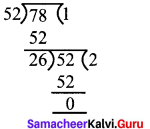26 is the HCF of 52 & 78Question 2.
Find HCF of 56 & 98 using repeated subtraction method.
Solution:
Let m = 98, n = 56
m – n = 98 – 56 = 42 ; now m = 56 & n = 42
m – n = 56 – 42 = 14 ; now m = 42 & n = 14
m – n = 42 – 14 = 28 ; now m = 14 & n = 28
m – n = 28 – 14 = 14 ; now = 14 & n = 14
∴ HCF of 98 & 56 is 14.

Question 3.
Find the first 10 terms of the Fibonacci series.
Solution:
Let us first write down the first 2 terms which are 1, 1. To find the remaining terms, we use the general formula. F(n) = F(n – 1) + F(n – 2)
∴ F(3) = F(2) + F(1)
F(1) = 1; F(2) = 1
F(3) = 1 + 1=2
F(4) = F(3) + F(2) = 2 + 1 = 3
F(5) = F(4) + F(3) = 2 + 3 = 5
∴ The first 10 terms are; 1, 1, 2, 3, 5, 8,13, 21, 34, 55

Question 4.
In a particular method of decoding, the word SECRET is written as TFDSFU. What is the cipher text for PASSWORD?
Solution:
Given that SECRET is written as TFDSFU. We notice that every letter is substituted by the next letter alphabetically.
So S is substituted by T
E by F, C by D, R by S E by F, & T by U
∴ for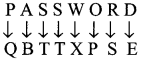similarly we have to substitute
∴ The cypher is QBTTXPSE.Question 5.
Using the Pigpen code, decode the below message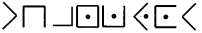Solution:
The pigpen code is generated by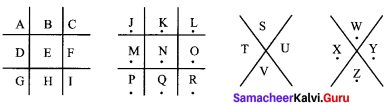By taking the outlines corresponding to each letter, we get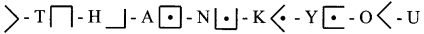∴ The message is THANK YOU

Question 6.
Using the reflection table given below, find the encoded message Reflection table.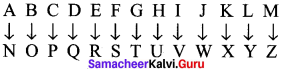Message: PBATENGHYNGVBAF
Solution:
To decode the above message, let us substitute the letters that correspond’to the message from the reflection table. So, we get
P → C
B → O
A → N
T → G
E → R
N → A
G → T
H → U
Y → L
N → A
G → T
V → l
B → O
A → N
F → S
The word is CONGRATULATIONS!